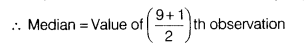# Find the median of the following data. 37, 31, 42, 43, 46, 25, 39, 45, 32

i) Find the median of the following data.
37, 31, 42, 43, 46, 25, 39, 45, 32 If 37 is replaced by 73, what will be the new median?
(ii) In the above data, if each term is increased by 2, then what is the effect on new median?

(i) First, arrange the given data in ascending order, as shown below
25, 31,32, 37, 39, 42, 43, 45, 46
Here, the number of observations, n = 9 (odd)= Value of 5th observation = 39
Now, if we replaced 37 by 73, then the new observations arranged in ascending order as shown below
25, 31,32, 39, 42, 43, 45, 46, 73
∴ New median = Value of 5th observation=42
(ii) Yes, their is effect on median while increasing each term by 2.